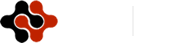International Journal of Electronic Engineering and Computer Science, Vol. 1, No. 1, August 2016 Publish Date: Aug. 19, 2016 Pages: 40-44

The Two Quantum Measurement Theories and the Bell-Kochen-Specker Paradox

1Department of Physics, Korea Advanced Institute of Science and Technology, Daejeon, Korea

2Department of Information and Computer Science, Keio University, Yokohama, Japan

Abstract

We review a property of a new measurement theory based on the truth values. The results of measurements are either 0 or 1. The measurement theory accepts a hidden variable model for a single Pauli observable. Therefore we can introduce a classical probability space for the measurement theory in this case. And we can measure the single Pauli observable by using the measurement theory based on the truth values. Our discussion provides a new insight to formulate quantum measurement theory, by using the measurement theory here based on the truth values. In this paper, we discuss the fact that the projective measurement theory (the results of measurements are either +1 or -1) says the Bell, Kochen, and Specker (BKS) paradox for the single Pauli observable. Therefore, we cannot introduce a classical probability space for the measurement theory in this case. Our discussion says that we cannot measure the single Pauli observable by using the projective measurement theory without the BKS paradox.

Keywords

Quantum Measurement Theory, Quantum Non Locality, Formalism

1. Introduction

The projective measurement theory (cf. [1-6]) gives at times remarkably accurate numerical predictions.

From the incompleteness argument of Einstein, Podolsky, and Rosen (EPR) , a hidden-variable interpretation of quantum mechanics has been an attractive topic of research [3, 4]. One is the Bell-EPR theorem . Another is the no-hidden-variable theorem of Kochen and Specker (the KS theorem) . Greenberger, Horne, and Zeilinger discover [10, 11] the so-called GHZ theorem for four-partite GHZ state. And, the Bell-KS theorem becomes very simple form (see also Refs. [12-16]).

The Leggett-type nonlocal hidden-variable theory  is experimentally investigated [18-20]. The experiments report that quantum mechanics does not accept the Leggett-type nonlocal hidden-variable theory. These experiments are performed in four-dimensional space (two parties) in order to study a nonlocality of the hidden-variable theory. However there are debates for the conclusions of the experiments. See Refs. [21-23].

For the applications of quantum mechanics, an implementation of a quantum algorithm to solve Deutsch’s problem [24-26] on a nuclear magnetic resonance quantum computer is reported firstly . An implementation of the Deutsch-Jozsa algorithm on an ion-trap quantum computer is also reported . There are several attempts to use single-photon two-qubit states for quantum computing. Oliveira et al. implement Deutsch’s algorithm with polarization and transverse spatial modes of the electromagnetic field as qubits . Single-photon Bell states are prepared and measured . Also the decoherence-free implementation of Deutsch's algorithm is reported by using such a single-photon and by using two logical qubits . More recently, a one-way based experimental implementation of Deutsch's algorithm is reported . In 1993, the Bernstein-Vazirani algorithm was reported . It can be considered as an extended Deutsch-Jozsa algorithm. In 1994, Simon's algorithm was reported . An implementation of a quantum algorithm to solve the Bernstein-Vazirani parity problem without an entanglement on an ensemble quantum computer is reported . A fiber-optics implementation of the Deutsch-Jozsa and Bernstein-Vazirani quantum algorithms with three qubits is discussed . A quantum algorithm for approximating the influences of Boolean functions and its applications is recently reported .

We review a property of a new measurement theory based on the truth values . The results of measurements are either 0 or 1. The measurement theory accepts a hidden variable model for a single Pauli observable. Therefore we can introduce a classical probability space for the measurement theory in this case. And we can measure the single Pauli observable by using the measurement theory based on the truth values. Our discussion provides new insight to formulate quantum measurement theory, by using the measurement theory based on the truth values.

In this paper, we discuss the fact that the projective measurement theory (the results of measurements are either +1 or -1) says the Bell, Kochen, and Specker (BKS) paradox for the single Pauli observable. Therefore, we cannot introduce a classical probability space for the measurement theory in this case. Our discussion says that we cannot measure the single Pauli observable by using the projective measurement theory without the BKS paradox.

2. The Measurement Theory Based on the Truth Values and a Hidden Variable Model

We review the new measurement theory meets a hidden variable model of a single spin observable. Assume a spin-1/2 state. Let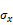be a single Pauli observable. We have a quantum expected value as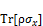(1)

We derive a necessary condition for the quantum expected value for the system in a spin-1/2 state given in (1). We have

0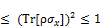(2)

It is worth noting here that we have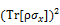=1 ifis the pure state lying in the x-direction. Hence we derive the following proposition concerning quantum mechanics when the system is in the state lying in the x-direction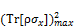=1               (3)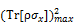is the maximal possible value of the product. It is worth noting here that we have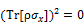when the system is in the pure state lying in the z-direction. Thus we have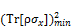=0              (4)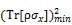is the minimal possible value of the product. In short, we have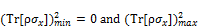=1  (5)

In what follows, we derive the above proposition (5) assuming the following form: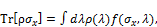(6)

where λ denotes some hidden variable and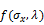is the hidden result of measurements of the Pauli observable. We assume that the values ofare either 1 or 0 (in ħ/2 unit).

Let us assume the hidden variable theory of the single spin observable based on the new measurement theory. In this case, the quantum expected value in (1), which is the average of the hidden results of the new measurements, is given by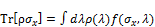(7)

The possible values of the hidden resultare either 1 or 0 (in ħ/2 unit). The same expected value is given by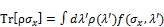(8)

because we only change the notation as λ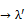. Of course, the possible values of the hidden result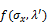are either 1 or 0 (in ħ/2 unit). By using these facts, we derive a necessary condition for the expected value for the system in the spin-1/2 state lying in the x-direction. We derive the possible values of the product. We have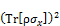=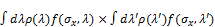=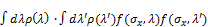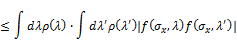=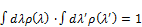(9)

Clearly, the above inequality can have the upper limit since the following cases are possible: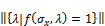=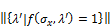(10)

and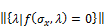=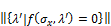(11)

Thus we derive a proposition concerning the hidden variable theory based on the new measurement theory (in a spin-1/2 system), that is,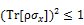. Hence we derive the following proposition concerning the hidden variable theory:=1                 (12)

We derive another necessary condition for the expected value for the system in the pure spin-1/2 state lying in the z-direction. We have==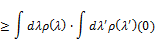=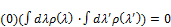(13)

Clearly, the above inequality can have the lower limit since the following cases are possible:=(14)

and=(15)

Thus we derive a proposition concerning the hidden variable theory based on the new measurement theory (in a spin-1/2 system), that is,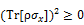. Hence we derive the following proposition concerning the hidden variable theory=0                  (16)

Thus from (12) and (16) we have=1      (17)

Clearly, we can assign the truth value "1" for the two propositions (5) (concerning quantum mechanics) and (17) (concerning the hidden variable theory based on the new measurement theory), simultaneously. Therefore, the new measurement theory meets the existence of the hidden variable theory of the single spin observable.

3. The Projective Measurement Theory and the BKS Theorem

We discuss the fact that the projective measurement theory (the results of measurements are either +1 or -1) says the Bell, Kochen, and Specker (BKS) paradox for the single Pauli observable. Therefore we cannot introduce a classical probability space for the measurement theory in this case. Our discussion says that we cannot measure the single Pauli observable by using the projective measurement theory without the BKS paradox.

In what follows, we cannot derive the proposition (5) assuming the following form:(18)

where λ denotes some hidden variable, andis the hidden result of measurements of the Pauli observable. We assume that the values ofare either +1 or -1 (in ħ/2 unit).

Let us assume a hidden variable model based on the projective measurement theory of the single spin observable. In this case, the quantum expected value in (1), which is the average of the hidden results of the projective measurements, is given by(19)

The possible values of the hidden resultare either +1 or -1 (in ħ/2 unit). The same expected value is given by(20)

because we only change the notation as λ. Of course, the possible values of the hidden resultare either +1 or -1 (in ħ/2 unit). By using these facts, we derive a necessary condition for the expected value for the system in the spin-1/2 state lying in the x-direction. We derive the possible values of the product. We have===(21)

Clearly, the above inequality can have the upper limit since the following cases are possible:=(22)

and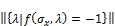=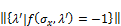(23)

Thus we derive a proposition concerning the hidden variable theory based on the projective measurement theory (in a spin-1/2 system), that is,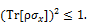Hence we derive the following proposition concerning the hidden variable theory=1             (24)

We derive another necessary condition for the expected value for the system in the pure spin-1/2 state lying in the z-direction.

We introduce an assumption that Sum rule and Product rule commute with each other . We do not pursue the details of the assumption. To pursue the details is an interesting point. It is suitable to the next step of researches. We have==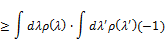=(25)

Clearly, the above inequality can have the lower limit since the following cases are possible:=(26)

and=(27)

Thus we derive a proposition concerning the hidden variable theory based on the projective measurement theory (in a spin-1/2 system), that is,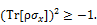Hence we derive the following proposition concerning the hidden variable theory=-1               (28)

Thus from (24) and (28) we have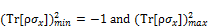=1    (29)

Clearly, we cannot assign the truth value "1" for two propositions (5) (concerning quantum mechanics) and (29) (concerning the hidden variable theory based on the projective measurement theory), simultaneously. In fact, we are in the BKS contradiction. Therefore, the projective measurement theory does not meet the existence of the hidden variable theory of the single spin observable.

4. Conclusions

In conclusions, we have reviewed a property of a new measurement theory based on the truth values. The results of measurements have been either 0 or 1. The measurement theory has accepted a hidden variable model for a single Pauli observable. Therefore we can have introduced a classical probability space for the measurement theory in this case. And we can measure the single Pauli observable by using the measurement theory based on the truth values. Our discussion has provided new insight to formulate quantum measurement theory, by using the measurement theory based on the truth values.

In this paper, we have discussed the fact that the projective measurement theory (the results of measurements are either +1 or -1) says the Bell, Kochen, and Specker (BKS) paradox for the single Pauli observable. Therefore we cannot introducea classical probability space for the measurement theory in this case. Our discussion has said that we cannot measure the single Pauli observable by using the projective measurement theory without the BKS paradox.

References

1. J. von Neumann, Mathematical Foundations of Quantum Mechanics (Princeton University Press, Princeton, New Jersey, 1955).
2. J. J. Sakurai, Modern Quantum Mechanics (Addison-Wesley Publishing Company, 1995), Revised ed.
3. A. Peres, Quantum Theory: Concepts and Methods (Kluwer Academic, Dordrecht, The Netherlands, 1993).
4. M. Redhead, Incompleteness, Nonlocality, and Realism (Clarendon Press, Oxford, 1989), 2nd ed.
5. M. A. Nielsen and I. L. Chuang, Quantum Computation and Quantum Information (Cambridge University Press, 2000).
6. R. P. Feynman, R. B. Leighton, and M. Sands, Lectures on Physics, Volume III, Quantum mechanics (Addison-Wesley Publishing Company, 1965).
7. A. Einstein, B. Podolsky, and N. Rosen, Phys. Rev. 47, 777 (1935).(Can Quantum-Mechanical Description of Physical Reality Be Considered Complete?)
8. J. S. Bell, Physics 1, 195 (1964).(On the Einstein–Podolsky–Rosen paradox).
9. S. Kochen and E. P. Specker, J. Math. Mech. 17, 59 (1967).(The problem of hidden variables in quantum mechanics).
10. D. M. Greenberger, M. A. Horne, and A. Zeilinger, in Bell's Theorem, Quantum Theory and Conceptions of the Universe, edited by M. Kafatos (Kluwer Academic, Dordrecht, The Netherlands, 1989), pp. 69-72.(Going beyond Bell’s theorem)
11. D. M. Greenberger, M. A. Horne, A. Shimony, and A. Zeilinger, Am. J. Phys. 58, 1131 (1990).(Bell’s Theorem without Inequalities)
12. C. Pagonis, M. L. G. Redhead, and R. K. Clifton, Phys. Lett. A 155, 441 (1991).(The breakdown of quantum non-locality in the classical limit).
13. N. D. Mermin, Phys. Today 43(6), 9 (1990).(What’s Wrong with These Elements of Reality?)
14. N. D. Mermin, Am. J. Phys. 58, 731 (1990).(Quantum mysteries revisited)
15. A. Peres, Phys. Lett. A 151, 107 (1990).(Incompatible results of quantum measurements)
16. N. D. Mermin, Phys. Rev. Lett. 65, 3373 (1990). (Simple Uniﬁed Form for the Major No-Hidden-Variables Theorems)
17. A. J. Leggett, Found. Phys. 33, 1469 (2003).(Nonlocal Hidden-Variable Theories and Quantum Mechanics: An Incompatibility Theorem)
18. S. Groblacher, T. Paterek, R. Kaltenbaek, C. Brukner, M. Zukowski, M. Aspelmeyer, and A. Zeilinger, Nature (London) 446, 871 (2007).(An experimental test of non-local realism)
19. T. Paterek, A. Fedrizzi, S. Groblacher, T. Jennewein, M. Zukowski, M. Aspelmeyer, and A. Zeilinger, Phys. Rev. Lett. 99, 210406 (2007).(Experimental test of non-local realistic theories without the rotational symmetry assumption)
20. C. Branciard, A. Ling, N. Gisin, C. Kurtsiefer, A. Lamas-Linares, and V. Scarani,Phys. Rev. Lett. 99, 210407 (2007).(Experimental falsification of Leggett's nonlocal variable model)
21. A. Suarez, Found. Phys. 38, 583 (2008).(Nonlocal "Realistic" Leggett Models Can be Considered Refuted by the Before-Before Experiment)
22. M. Zukowski, Found. Phys. 38, 1070 (2008).(Comment on: Nonlocal "Realistic" Leggett Models Can be Considered Refuted by the Before-Before Experiment)
23. A. Suarez, Found. Phys. 39, 156 (2009).(On Bell, Suarez-Scarani, and Leggett Experiments: Reply to a Comment by Marek Zukowski in [Found. Phys. 38:1070, 2008])
24. D. Deutsch, Proc. Roy. Soc. London Ser. A 400, 97 (1985).(Quantum theory, the Church-Turing principle and the universal quantum computer)
25. D. Deutsch and R. Jozsa, Proc. Roy. Soc. London Ser. A 439, 553 (1992).(Rapid Solution of Problems by Quantum Computation)
26. R. Cleve, A. Ekert, C. Macchiavello, and M. Mosca, Proc. Roy. Soc. London Ser. A 454, 339 (1998).(Quantum algorithms revisited)
27. J. A. Jones and M. Mosca, J. Chem. Phys. 109, 1648 (1998).(Implementation of a quantum algorithm on a nuclear magnetic resonance quantum computer)
28. S. Gulde, M. Riebe, G. P. T. Lancaster, C. Becher, J. Eschner, H. Haffner, F. Schmidt-Kaler, I. L. Chuang, and R. Blatt, Nature (London) 421, 48 (2003).(Implementation of the Deutsch-Jozsa Algorithm on an Ion-Trap Quantum Computer)
29. A. N. de Oliveira, S. P. Walborn, and C. H. Monken, J. Opt. B: Quantum Semiclass. Opt. 7, 288-292 (2005).(Implementing the Deutsch algorithm with polarization and transverse spatial modes)
30. Y.-H. Kim, Phys. Rev. A 67, 040301(R) (2003).(Single-Photon Two-Qubit Entangled States: Preparation and Measurement)
31. M. Mohseni, J. S. Lundeen, K. J. Resch, and A. M. Steinberg, Phys. Rev. Lett. 91, 187903 (2003).(Experimental Application of Decoherence-Free Subspaces in an Optical Quantum-Computing Algorithm)
32. M. S. Tame, R. Prevedel, M. Paternostro, P. Bohi, M. S. Kim, and A. Zeilinger,Phys. Rev. Lett. 98, 140501 (2007).(Experimental realization of Deutsch’s algorithm in a one-way quantum computer)
33. E. Bernstein and U. Vazirani, Proceedings of the Twenty-Fifth Annual ACM Symposium on Theory of Computing (STOC '93), pp. 11-20 (1993), doi:10.1145/167088.167097; SIAM J. Comput. 26-5, pp. 1411-1473 (1997).(Quantum complexity theory)
34. D. R. Simon, Foundations of Computer Science, (1994) Proceedings., 35th Annual Symposium on: 116-123, retrieved 2011-06-06.(On the power of quantum computation)
35. J. Du, M. Shi, X. Zhou, Y. Fan, B. J. Ye, R. Han, and J. Wu, Phys. Rev. A 64, 042306 (2001). (Implementation of a quantum algorithm to solve the Bernstein-Vazirani parity problem without entanglement on an ensemble quantum computer)
36. E. Brainis, L.-P. Lamoureux, N. J. Cerf, P. Emplit, M. Haelterman, and S. Massar,Phys. Rev. Lett. 90, 157902 (2003).(Fiber-optics implementation of the Deutsch-Jozsa and Bernstein-Vazirani quantum algorithms with three qubits)
37. H. Li and L. Yang, Quantum Inf. Process. 14, 1787 (2015). (A quantum algorithm for approximating the influences of Boolean functions and its applications)
38. K. Nagata and T. Nakamura, Int. J. Theor. Phys. 55, 3616, (2016). (Measurement Theory in Deutsch’s Algorithm Based on the Truth Values)
39. K. Nagata and T. Nakamura, Physics Journal, Volume 1, Issue 3, 183 (2015). (On the Bell-Kochen-Specker paradox)

 Contents 1. 2. 3. 4.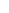600 ATLANTIC AVE, BOSTON,
MA 02210, USA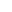+001-6179630233
JournalsJournal ListsAuthor GuidelinesPayment InformationSubmit a ManuscriptOpen Special IssuesJoin as Editorial MembersJoin as ReviewersPrivacy NoticeTerms of ConditionsHelp Center